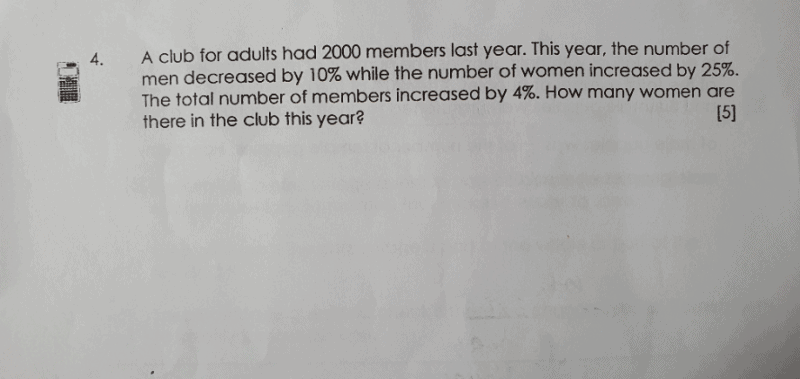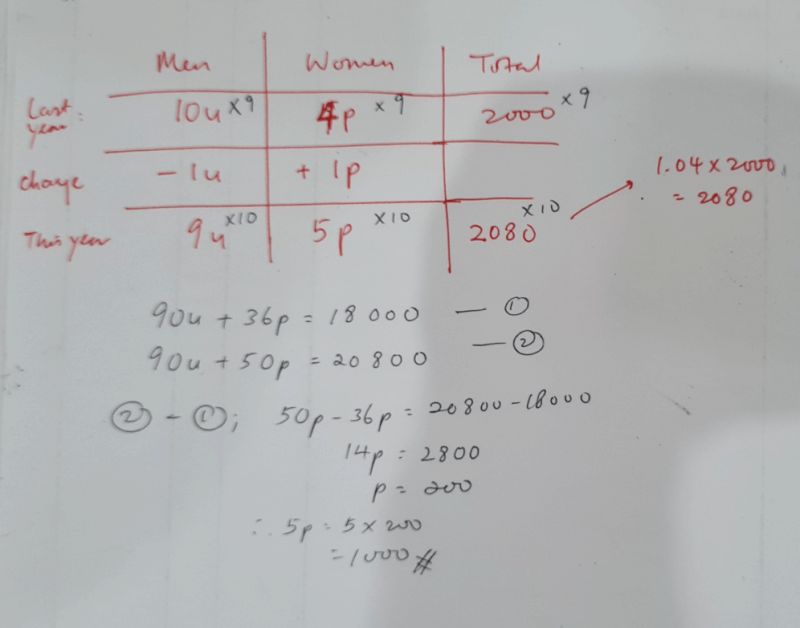# QuestionCan someone help 🙁 stuck for very long.

Let number of men be x and let number of women be y for the previous year

x + y = 2000 – (1)

Men decreased by 10% and women increased by 25%, total increase by 4%.

1.04(2000)=2080

Therefore,

0.9x + 1.25y = 2080 -(2)

From (1), taking 0.9(1),

0.9x + 0.9y = 0.9(2000) = 1800 – (3)

(2) – (3)

0.35y = 280

y = 280/0.35 = 800

number of women in the club this year = 800(1.25) = 1000 (Ans)

0 Replies 1 Like0 Replies 1 Like

Let men be units and women be parts.

10% = 1/10 (10u men last year, 9u this year)

25% = 1/4 (4p women last year, 5p this year)

104/100 x 2000 = 2080 (total number this year)

10u + 4p = 2000      x5

9u + 5p = 2080        x4

Make parts the same.

50u + 20p = 10000

36u + 20p = 8320

50u – 36u = 14u

10000 – 8320 = 1680

14u = 1680

9u = 1680/14 x 9

= 1080 (total men this year)

2080 – 1080 = 1000

0 Replies 1 Like